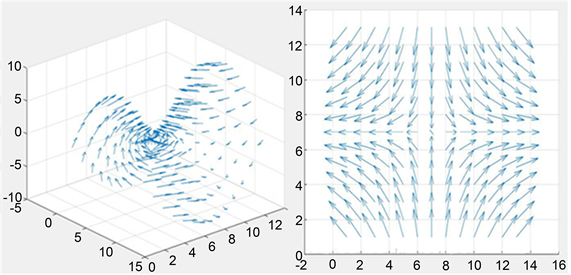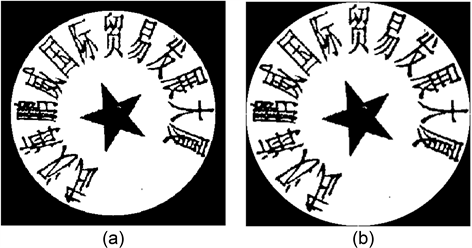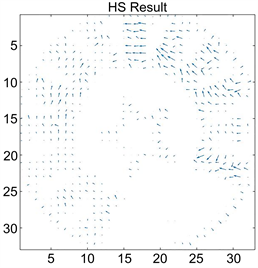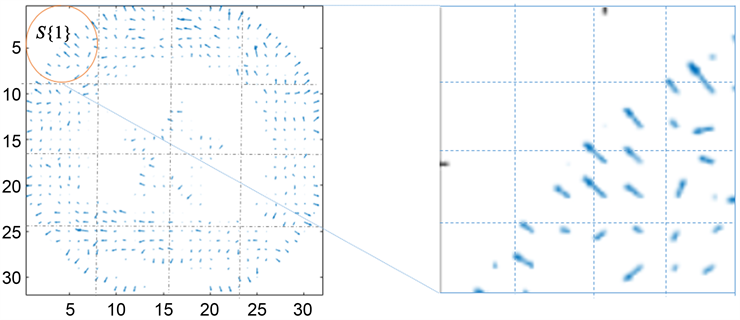# 基于Horn-Schunck光流运动矢量法的印章图像匹配Seal Image Matching Based on Horn-Schunck Optical Flow Motion Vector Method

DOI: 10.12677/CSA.2020.103054, PDF, HTML, XML, 下载: 172  浏览: 397

Abstract: The HS optical flow method is one of the mainstream methods of moving target methods based on machine vision. In 1981, the optical flow constraint equation was proposed by Horn and Schunck et al., which laid a deep foundation for optical flow calculation. In this paper, by introducing the HS optical flow calculation method, the optical flow velocity vector field of the seal to be identified and the archived seal is generated, and the local variance of the vector error is calculated to com-prehensively determine the authenticity of the seal to be identified. The experimental results prove that the HS optical flow method can discriminate authentic seals to a certain extent.

1. 引言

1976年，Pogglo和Reichartdt提出了关于光流的粗略计算形式；1981年，Horn和Schunck、Lucas提出了有效计算方法，引入了光流约束方程，对光流计算奠定了深厚基础。随后，光流被广泛运用于图像处理、目标跟踪等领域，研究人员将这些新方法分为五类 ：基于梯度的方法、基于匹配的方法、基于相位的方法、基频域的方法、基于神经动力学的方法。

2. 光流法的基本原理

2.1. 光流场与运动场Figure 1. 3D space motion vector field and 2D space motion vector field

2.2. 基本光流约束方程

$I\left(x+\text{d}x,y+\text{d}y,t+\text{d}t\right)=I\left(x,y,t\right)$

$I\left(x,y,t\right)+\frac{\partial I}{\partial x}\text{d}x+\frac{\partial I}{\partial y}\text{d}y+\frac{\partial I}{\partial t}\text{d}t+o\left({\partial }^{2}\right)=I\left(x,y,t\right)$

${I}_{x}u+{I}_{y}v+{I}_{t}=0$

2.3. 光流法的优劣

3. HS光流法

Horn-Schunck光流法是在光照强度不变的假设条件下  ，引入了全局光流平滑约束。假设物体运动矢量是平滑的或是缓慢变化的，即整个图像上的光流变化是平滑的，相邻像素点间的速度变化是相同的。Horn与Schunck引入光流基本约束方程和全局光流平滑约束来计算V，由于图像相邻像素点间的运动速度相同，因此速度的空间变化率为0，而得到光流矢量约等于0。对于图像的局部区域来说，引入光流平滑约束的的基本前提就是光流要尽可能的平滑，使得平滑约束项极小化。

${E}_{s}=\iint {\left[{\left(\frac{\partial u}{\partial x}\right)}^{2}+{\left(\frac{\partial u}{\partial y}\right)}^{2}+{\left(\frac{\partial v}{\partial x}\right)}^{2}+{\left(\frac{\partial v}{\partial y}\right)}^{2}\right]}^{2}\text{d}x\text{d}y$

${E}_{c}=\iint {\left({I}_{x}u+{I}_{y}v+{I}_{t}\right)}^{2}\text{d}x\text{d}y$

$L=\iint \left\{{\left({I}_{x}u+{I}_{y}v+{I}_{t}\right)}^{2}+\gamma {\left[{\left(\frac{\partial u}{\partial x}\right)}^{2}+{\left(\frac{\partial u}{\partial y}\right)}^{2}+{\left(\frac{\partial v}{\partial x}\right)}^{2}+{\left(\frac{\partial v}{\partial y}\right)}^{2}\right]}^{2}\right\}\text{d}x\text{d}y=\mathrm{min}$

$L=\iint \left\{{\left({I}_{x}u+{I}_{y}v+{I}_{t}\right)}^{2}+\gamma {\left[{\left(\frac{\partial u}{\partial x}\right)}^{2}+{\left(\frac{\partial u}{\partial y}\right)}^{2}+{\left(\frac{\partial v}{\partial x}\right)}^{2}+{\left(\frac{\partial v}{\partial y}\right)}^{2}\right]}^{2}\right\}\text{d}x\text{d}y=\mathrm{min}$

${L}_{v}-\frac{\partial {L}_{vt}}{\partial t}-\frac{\partial {L}_{vx}}{\partial x}\frac{\partial {L}_{vy}}{\partial y}\frac{\partial {L}_{uz}}{\partial z}=0$

${I}_{x}^{2}u+{I}_{x}{I}_{y}v={a}^{2}{\nabla }^{2}u-{I}_{x}{I}_{t}$

${I}_{y}^{2}v+{I}_{x}{I}_{y}u={a}^{2}{\nabla }^{2}v-{I}_{y}{I}_{t}$

$\left\{\begin{array}{c}{I}_{x}\left({I}_{x}{u}^{n+1}+{I}_{t}\right)=-\gamma {\nabla }^{2}u\\ {\nabla }^{2}u={u}^{n+1}-{u}^{-n}\end{array}\right\}\to {I}_{x}^{2}{u}^{n+1}+{I}_{x}{I}_{y}{v}^{n+1}+{I}_{x}{I}_{t}=-\gamma {u}^{n+1}+\gamma {u}^{-n}$

$\left\{\begin{array}{c}{I}_{y}\left({I}_{x}{u}^{n+1}+{I}_{t}\right)=-\gamma {\nabla }^{2}v\\ {\nabla }^{2}v={v}^{n+1}-{v}^{-n}\end{array}\right\}\to {I}_{y}^{2}{v}^{n+1}+{I}_{x}{I}_{y}{u}^{n+1}+{I}_{y}{I}_{t}=-\gamma {v}^{n+1}+\gamma {v}^{-n}$

${u}^{n+1}={u}^{-n}-\frac{{I}_{x}\left({I}_{x}{u}^{-n}+{I}_{t}+{I}_{y}{v}^{-n}\right)}{\gamma +\left({I}_{x}^{2}+{I}_{y}^{2}\right)}$

${v}^{n+1}={v}^{-n}-\frac{{I}_{y}\left({I}_{x}{u}^{-n}+{I}_{t}+{I}_{y}{v}^{-n}\right)}{\gamma +\left({I}_{x}^{2}+{I}_{y}^{2}\right)}$

$\stackrel{¯}{{u}_{i,j,k}}=\frac{1}{6}\left({u}_{i,j-1,k}+{u}_{i-1,j,k}+{u}_{i,j+1,k}+{u}_{i+1,j,k}\right)+\frac{1}{12}\left({u}_{i-1,j,k}+{u}_{i-1,j+1,k}+{u}_{i+1,j+1,k}+{u}_{i+1,j-1,k}\right)$

$\stackrel{¯}{{v}_{i,j,k}}=\frac{1}{6}\left({u}_{i,j-1,k}+{u}_{i-1,j,k}+{u}_{i,j+1,k}+{u}_{i+1,j,k}\right)+\frac{1}{12}\left({u}_{i-1,j,k}+{u}_{i-1,j+1,k}+{u}_{i+1,j+1,k}+{u}_{i+1,j-1,k}\right)$

${I}_{x}=\frac{1}{4}\left({I}_{i,j+1,k}-{I}_{i,j,k}+{I}_{i+1,j+1,k}-{I}_{i+1,j,k}+{I}_{i,j+1,k+1}-{I}_{i,j,k+1}+{I}_{i+1,j+1,k+1}-{I}_{i+1,j,k+1}\right)$

${I}_{y}=\frac{1}{4}\left({I}_{i+1,j,k}-{I}_{i,j,k}+{I}_{i+1,j+1,k}-{I}_{i,j+1,k}+{I}_{i+1,j,k+1}-{I}_{i,j,k+1}+{I}_{i+1,j+1,k+1}-{I}_{i,j+1,k+1}\right)$

${I}_{t}=\frac{1}{4}\left({I}_{i,j,k+1}-{I}_{i,j,k}+{I}_{i,j+1,k+1}-{I}_{i,j+1,k}+{I}_{i+1,j,k+1}-{I}_{i+1,j,k}+{I}_{i+1,j+1,k+1}-{I}_{i+1,j+1,k}\right)$

4. HS光流算法实验

HS光流算法的执行步骤总结如下：

1) 读取待识别图像与参考图像数据并初始化矩阵；

2) 初始化迭代参数、平滑约束权重系数；

3) 计算灰度梯度 ${I}_{x}$${I}_{y}$${I}_{t}$，进行微分；

4) 计算 $k-1$ 次速度平均值；

5) 计算k次迭代的速度值；

6) 计算两次迭代的光流值误差，若小于给定误差值域则计算结束，否则进行 $k+1$ 次迭代。Figure 2. (a) Binary image of the stamp to be identified after morphological processing; (b) Binary image of archive stamp after morphological processingFigure 3. HS optical flow motion vector field

$\text{rate}=\frac{16-\text{count}}{16}$Table 1. Schematic classification of interval variance comparison resultsFigure 4. Optical flow field blocking effect diagram

$SumP=\underset{i=1}{\overset{16}{\sum }}{P}_{i},SumL=\underset{i=1}{\overset{16}{\sum }}{L}_{i}$

$Sumrate=\left(1-\frac{SumP}{SumP+SumL}\right)\ast 100$

5. 结论

  Horn, B.K.P. and Schunck, B.G. (1981) Determining Optical Flow. https://doi.org/10.1016/0004-3702(81)90024-2  朱璐瑶. 改进的Horn-Schunck光流法在目标追踪中的应用[J]. 中国体视学与图像分析, 2015, 20(3): 218-226.  李秀丽, 李旭健, 高林. 一种改进的视频运动目标检测算法[J]. 软件导刊, 2016, 15(8): 26-29.  Okamura, H. and Fujii, Y. (1988) Lorentzian Force Type Fibre-Optic AC Magnetic Sensor Realising Frequency-Independent Sensitivity. Electronics Letters, 24, 1090-1091. https://doi.org/10.1049/el:19880739  Wang, F.F., Han, J.L. and Guo, L.J. (2020) Optical Coherence Tomography and Fractional Flow Reserve Guided Treatment of Woven Coronary Artery Anomaly Presenting as Acute Myocardial Infarction: A Case Report. Medicine, 99, e19163. https://doi.org/10.1097/MD.0000000000019163  Meng, X., Gao, X.Y. and Du, Y.L. (2020) Influence of O2/Ar Flow Ratio on the Structure, Optical and Electrical Properties of DC Reactively Magnetron-Sputtered Face-Centered Cubic NiO Films at Room Temperature. Physica B: Physics of Condensed Matter, 579, Article ID: 411877. https://doi.org/10.1016/j.physb.2019.411877  Ramaiah, J., Ajithaprasad, S., Gannavarpu, R. and Ambrosini, D. (2020) Fast and Robust Method for Flow Analysis Using GPU Assisted Diffractive Optical Element Based Background Oriented Schlieren (BOS). Optics and Lasers in Engineering, 126, Article ID: 105908. https://doi.org/10.1016/j.optlaseng.2019.105908  Yoshitaka, F. and Takeharu, S. (2020) The Effect of Optical Flow Motion Direction on Vection Strength. i-Perception, 11. https://doi.org/10.1177/2041669519899108  Yoshinori, K., Tomoyo, S., Masahiro, H., Eisuke, U., Rikuta, H., Yoshihisa, K., Tadashi, M., Tetsumin, L., Taishi, Y. and Tsunekazu, K. (2020) Optical Coherence Tomogra-phy-Defined Plaque Vulnerability in Relation to Functional Stenosis Severity Stratified by Fractional Flow Reserve and Quantitative Flow Ratio. Catheterization and Cardiovascular Interventions: Official Journal of the Society for Cardiac Angiography & Interventions. https://doi.org/10.1002/ccd.28756  Priya, D., Pascal, A., Charuta, F., Berenice, F.I., Stephan, J., Khokha, M.K., Kahle, K.T. and Engin, D. (2020) Author Correction: Visualizing Flow in an Intact CSF Network Using Optical Coherence Tomography: Implications for Human Congenital Hydrocephalus. Scientific Reports, 10, Article No. 2791. https://doi.org/10.1038/s41598-020-59301-y